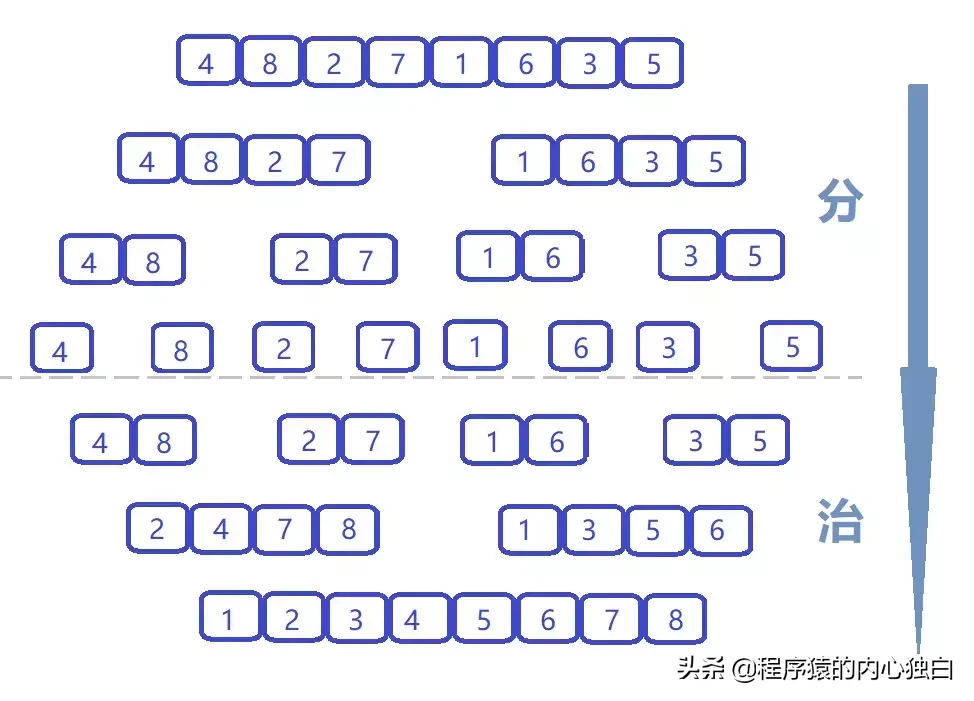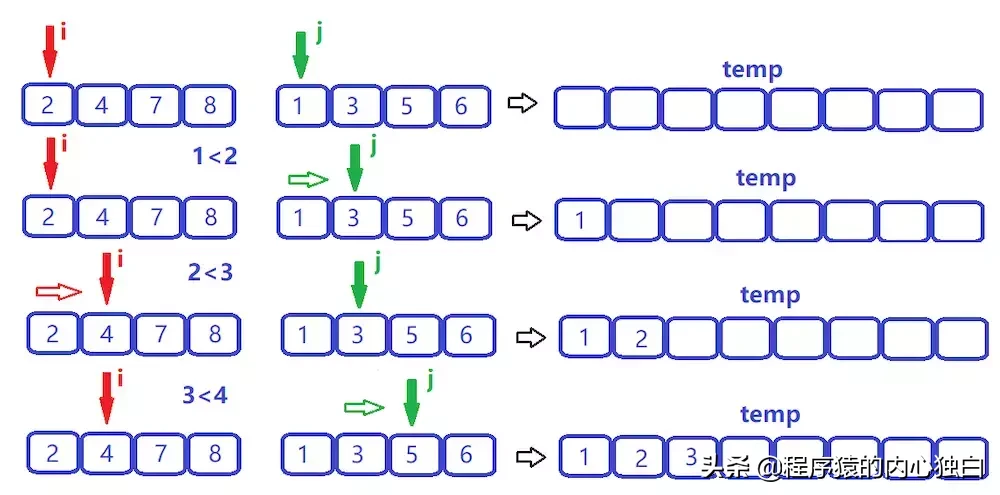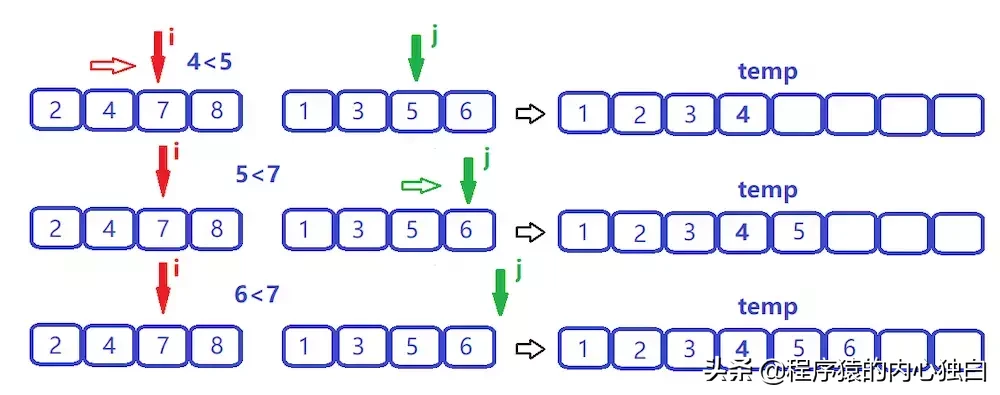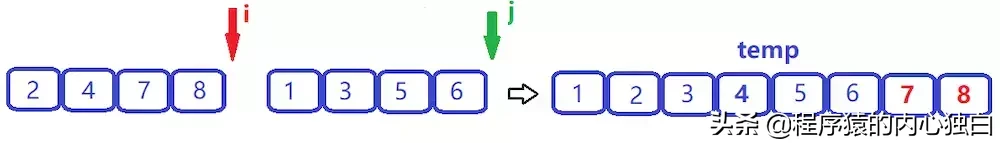# 归并排序

• 基本思路• 分而治之

• 归并操作
• 归并操作需要将两个已经有序的子序列合并成一个有序序列，我们选择图中的{2，4，7，8}{1，3，5，6}为例，它是怎么合并成一个有序数列呢？
• 我们先重新申请一个能容纳两个数组归并的空间(命名temp的数组)，两个哨兵指针 i 和 j 分别指向两个数组的开头；我们对比两个指针所指向数据的大小，将小的数据移向temp数组，指向该数据的指针向后移动。JAVA 代码

package DataStructure;

public class MergeSort {

public static void sort(int[] arr, int low, int high, int[] temp) {

if (low < high) {

int mid = (low + high) / 2;

sort(arr, low, mid, temp);

sort(arr, mid + 1, high, temp);

merge(arr, low, mid, high, temp); // 对两个子数组进行合并操作

}

}

private static void merge(int[] arr, int low, int mid, int high, int[] temp) {

int tempPoint = 0; // temp数组指针

int i = low; // i指针

int j = mid + 1; // j指针

while (i <= mid && j <= high)

if (arr[i] <= arr[j])

temp[tempPoint++] = arr[i++];

else

temp[tempPoint++] = arr[j++];

while (i <= mid)

temp[tempPoint++] = arr[i++]; // 左边剩余的填充至temp数组中

while (j <= high)

temp[tempPoint++] = arr[j++]; // 右边剩余的填充至temp数组中

int t = 0;

while (low <= high) // 返回至原数组

arr[low++] = temp[t++];

}

public static void main(String[] args) {

int[] arr = { 4, 8, 2, 7, 1, 6, 3, 5 };

int[] temp = new int[arr.length];

sort(arr, 0, arr.length - 1, temp);

for (int n : arr)

System.out.print(n + " ");

}

}

• 时间复杂度